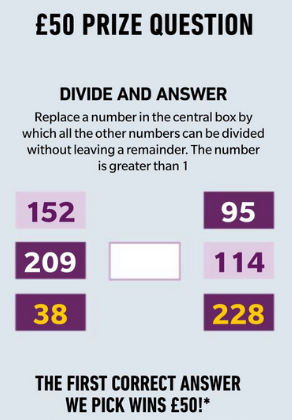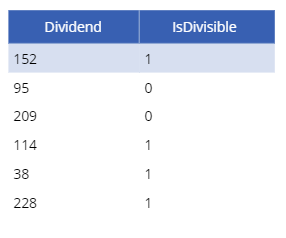# Walkthrough - Solving maths puzzles with Power Apps

For learning purposes, it can be useful to see a practical example of how to carry out mathematical tasks with Power Apps. This post walks through a demonstration of how to answer a quiz question using Power FX functions that include ForAll, Sequence, and Mod.

The background for this post comes from a question from a friend who wanted some help in answering a quiz question.

The image beneath describes the question - what number can be fully divided into 95, 114, 228, 152, 209, and 38 without any remainder?We can answer this type of question with almost all programming languages and in this post, I'll describe one technique to answer this question with Power Apps.

## How to find a number that can divide fully into several input values

The high-level methodology of a technique to solve this problem is to build a sequence of divisors (for example, 1-2000), and to attempt to divide each divisor by all the required dividends (152,95,209,114,38,228).

To implement this technique, we would call the ForAll function to iterate over the sequence of divisors. From within each iteration, we can test whether the divisor is divisible by all required dividends. If so, we can record the divisor that works. The formula beneath shows the formula that provides the answer to the question.

`With({targetDividends:RenameColumns([152,95,209,114,38,228],                                    "Value",                                     "Dividend"                       )      },     ForAll(Sequence(2000),           If(Sum(                  AddColumns(targetDividends,                              "IsDivisible",                             If(Mod(Dividend,Value)=0,1,0)                            ),                   IsDivisible               )=6,               Collect(colResults, {Dividend:Value})           )     ))`

## How the formula works

Let's take a closer look at how this formula works. From within the With block, we define a single-column table called targetDividends. The table contains a single column called "Dividend" and the rows in this table contains the values 152,95,209,114,38,228.

The ForAll function iterates over a sequence of values that range from 1-2000. We create this sequence by calling the Sequence function.

From within each iteration, we specify a formula that adds a column to the targetDividends table. The column we add is called "IsDivisible" and we set the value to the result of whether the dividend is divisible by the current sequence value in the iteration. We call the Mod function to determine if the dividend is fully divisible by the divider. The Mod function returns the remainder following the division of 2 input values.

As an illustration, the output of the AddColumns function for Sequence value 2 is a table that looks like this:We can then Sum the "IsDivisible" column and if the result is 6 (the target number of dividends), it means that the sequence value is divisible by all the dividends.

When this condition equals true, we call the Collect function to store the value in a collection called colResult.

The image below illustrates the result of this formula, and we can see that the possible answer to this question is 1 and 19. Since the instructions exclude 1 as a valid answer, 19 is the answer to this question.## Conclusion

It can be very useful to walk through the process of how to solve a mathematical problem with Power Apps, and this post gave an example of how to answer a quiz question by applying functions that include ForAll, Sequence, and Mod.